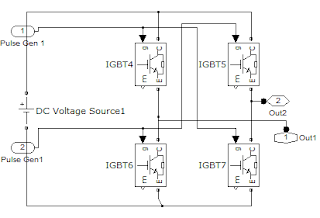Hello friends, After such a long break, I want to share a Matlab/Simulink model which I made for simulating 9 level cascaded multilevel inverter as mentioned in the title of this post. To be precise, this post deals with Single Phase Multilevel Inverter.

Usually the objective of multilevel inverter designers is to get a purely sinusoidal wave out of a Constant or variable DC Voltage source or current source. The inverters using DC Voltage Source are called VSI i.e. Voltage Source Inverters while the inverters made using Current source are called CSI i.e. Current Source Inverter. The circuit for both the types of inverters are basically same. Only difference is the presence of an extra inductor present in CSI.

In this post, I want to discuss only about Voltage Source Inverter (VSI). Using VSI, we can obtain a square wave output of 1 level or we can obtain an output of upto 2 level by making the changes in switch firing delay.

But if we want to get a purely sinusoidal wave, then it’s difficult (or probably impossible) with simple H-bridge based single phase inverter. For this reason, we use the concepts of multilevel inverters wherein the whole inverter system combined together will give an output in the form of steps which looks similar to sine wave inverters. Using large number of levels, by adjusting the delays and at the end by using a proper capacitor, we can get a purely sinusoidal wave.

As per my knowledge ‘APC’ Inverters by “Schneider Electric”, is one of the best inverter which produces a purely sinusoidal waveform.

Basically there are 3 topology of Multilevel Inverters

1.    Diode Clamped Multilevel Inverters
2.    Flying Capacitors Multilevel Inverters

In my model, I have used the Cascaded multilevel Inverter topology which is far better than others when cost of implementation is concerned. Using this topology, we can obtain n level using (n-1) H-bridges, with each bridge consisting of 4 switches like MOSFET, IGBT, etc.

Here, I have used 8 H-bridges Single phase Inverters and connected them in series.  By varying the switching timing in the ‘pulse generator’ block, I obtained 9 levels or steps in the output.

The Simulink block diagram is shown below:Complete Simulink model of the Inverter

I have created subsystem for each H-bridge and used the subsystems to avoid clutter in the workspace. The models present inside each H-bridge is shown in the figure given below:Subsystem of H-Bridge Inverter Blocks

The 9 level voltage output obtained is shown in the figure given below:Output Waveforms
The comparison between the sinusoidal wave and the obtained output can be observed by looking at the image. It can be inferred that the output is nearly close to the sinusoidal wave, but a lot can be done to improve. If the pulse width is adjusted properly, a nearly perfect sine wave can be obtained.

Also Read: 3 Phase Inverter in 180 degree conduction Mode Simulation in Simulink

If you have any query/doubt/suggestions/constructive criticism, then do comment below and let me know.

1.You share very awesome information in your article i like your post keep it up Good job

2.Valuable information. Thanks. But could you plsss tell me how you have selected the switching time instants. Plss repy .

3.nice and well easy to understand

4.hey i need the cascaded h-bridge inverter using pod pwm strategy..

5.this is a great jop ......my final year project is 7-level cascaded multilevel inverter ...and i have problem in the matlab simulation .....can you show the steps of simulation of your project step by step please...because i am new in matlab simulation ...and this is my e mail ....mustafa.7lfawi2015@hotmail.com

6.this is a great jop ......my final year project is 7-level cascaded multilevel inverter ...and i have problem in the matlab simulation .....can you show the steps of simulation of your project step by step please...because i am new in matlab simulation ...and this is my e mail ....mustafa.7lfawi2015@hotmail.com

7.i want to add current supression to this circuit can anyone solve my query

8.hello,
i you request the three phase NPC and cascade full bridge and FC inverter topology in matlab simulink, i need it pleaase, my email: kharrich.mohammed@gmail.com

9.Hello sir,

can you please explain that how to adjust the pulse width for the above example

10.sir,

i have generated 7 level using only 5 swithes.

m:- 8488894903

11.sir,

i have generated 7 level using only 5 swithes.

12.sir,

i have generated 7 level using only 5 swithes.

m:- 8488894903

13.Hi sir,
My name is babu. I am doing same project and i got 19% THD. so please tell me how to minimize THD. my mail id jbabu65@gmail.com.
thank you sir

14.do you have an email? ur design has an error while I run. thnx

15.I want the seven level multilevel invert 3 phase simulink

16.sir,
iam not getting output when iam connecting load

17.Sir can I know the phase given in different pulse generator?

18.Sir plz tell the phase of each pulse generator?

19.Sir can I know the phase given in different pulse generator?

If you liked this blog then Join me at: